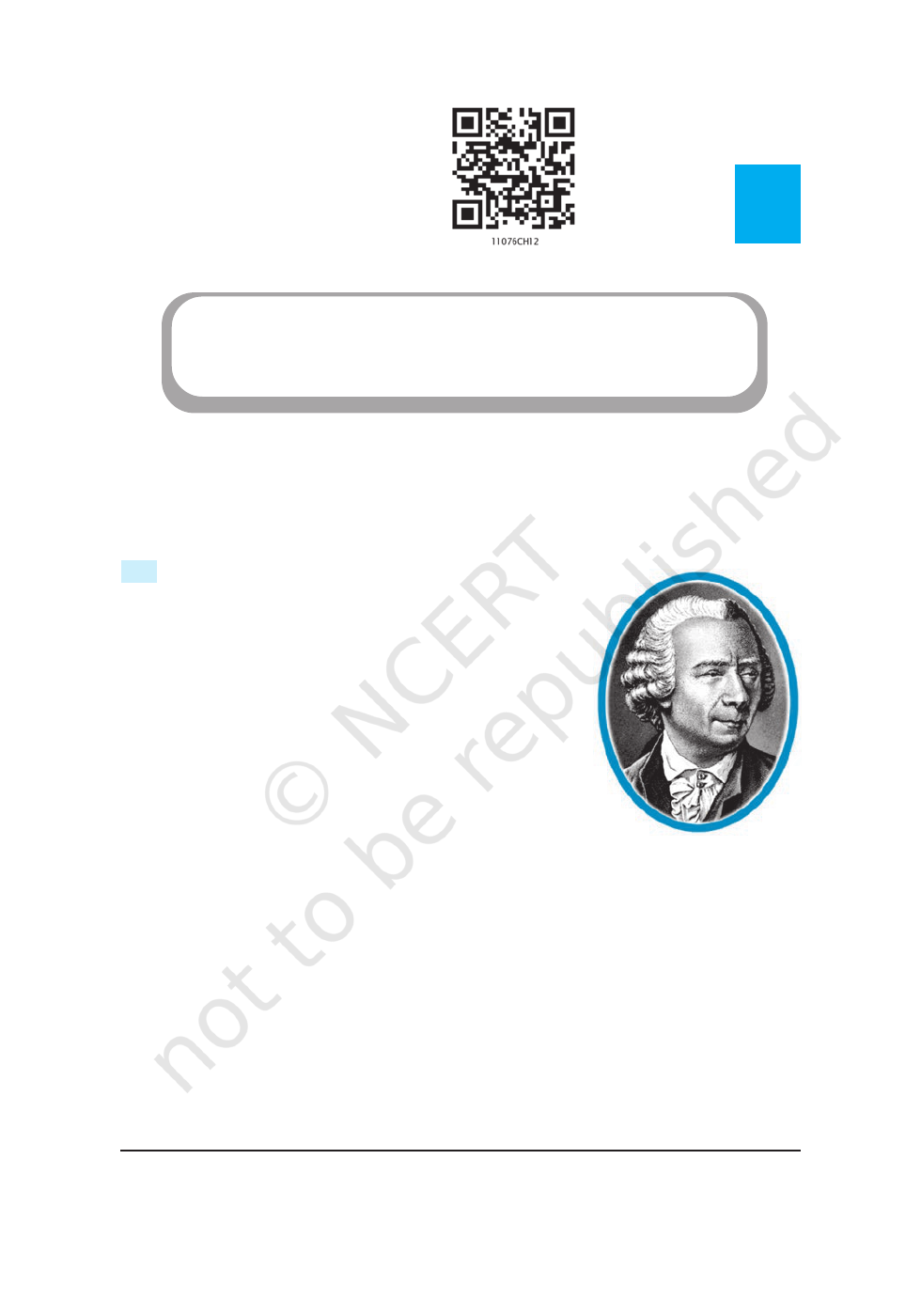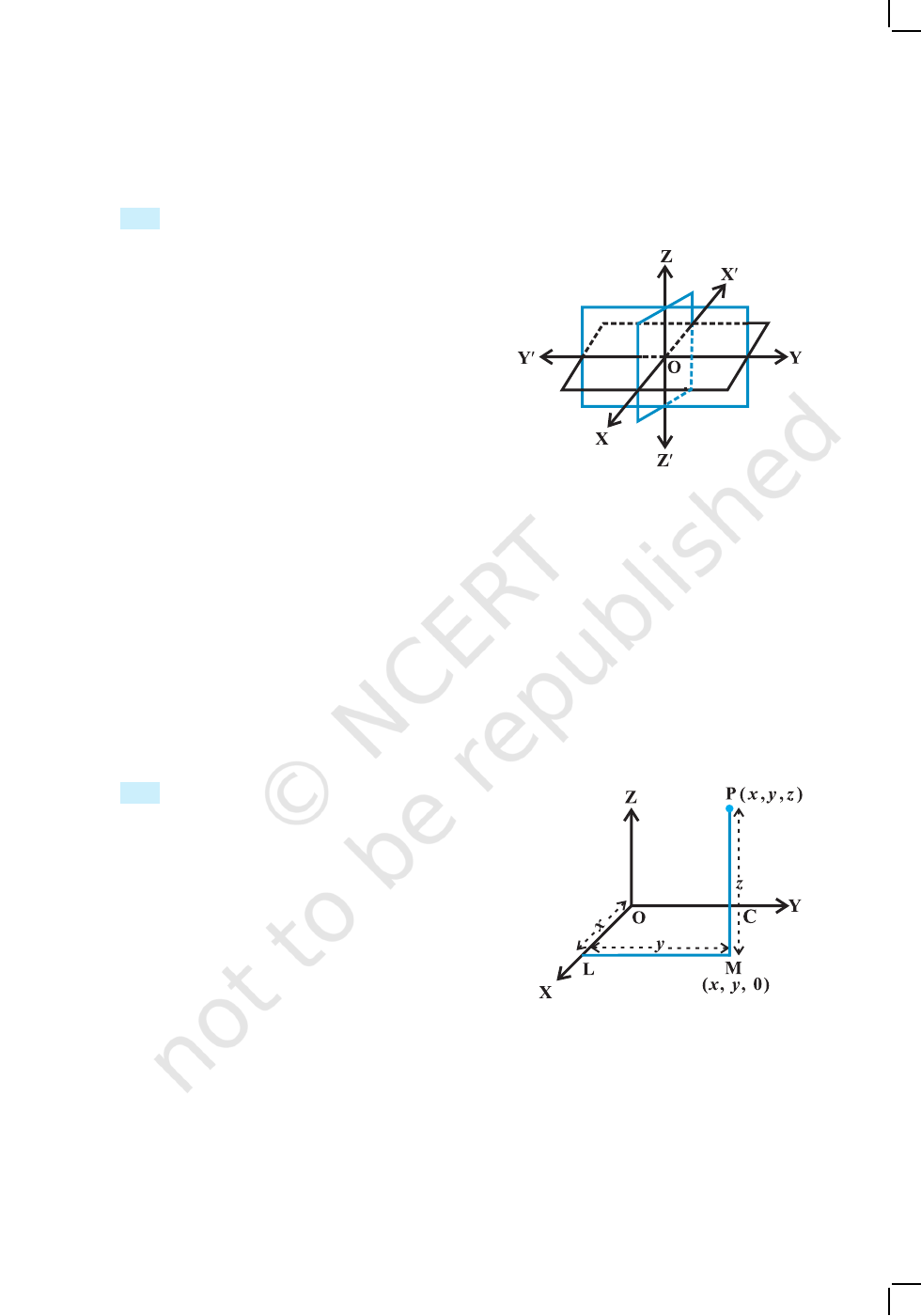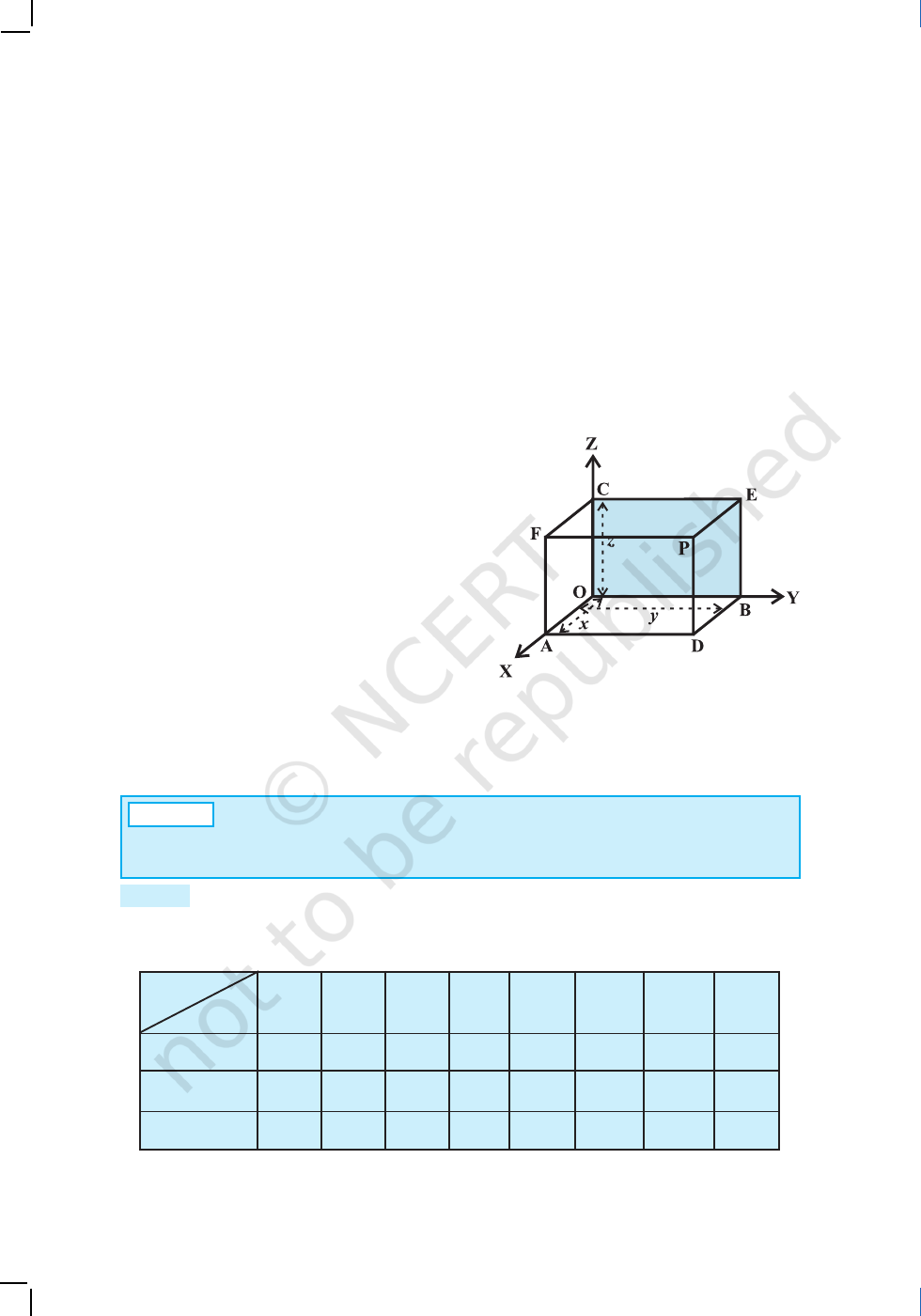vMathematics is both the queen and the hand-maiden of
all sciences – E.T. BELLv
12.1 Introduction
You may recall that to locate the position of a point in a
plane, we need two intersecting mutually perpendicular lines
in the plane. These lines are called the coordinate axes
and the two numbers are called the coordinates of the
point with respect to the axes. In actual life, we do not
have to deal with points lying in a plane only. For example,
consider the position of a ball thrown in space at different
points of time or the position of an aeroplane as it flies
from one place to another at different times during its flight.
Similarly, if we were to locate the position of the
lowest tip of an electric bulb hanging from the ceiling of a
room or the position of the central tip of the ceiling fan in a room, we will not only
require the perpendicular distances of the point to be located from two perpendicular
walls of the room but also the height of the point from the floor of the room. Therefore,
we need not only two but three numbers representing the perpendicular distances of
the point from three mutually perpendicular planes, namely the floor of the room and
two adjacent walls of the room. The three numbers representing the three distances
are called the coordinates of the point with reference to the three coordinate
planes. So, a point in space has three coordinates. In this Chapter, we shall study the
basic concepts of geometry in three dimensional space.*
*
For various activities in three dimensional geometry one may refer to the Book, “A Hand Book for
designing Mathematics Laboratory in Schools”, NCERT, 2005.
Leonhard Euler
(1707-1783)
12Chapter
INTRODUCTION TO THREE
DIMENSIONAL GEOMETRY
2020-21INTRODUCTION TO THREE DIMENSIONAL GEOMETRY 269
12.2 Coordinate Axes and Coordinate Planes in Three Dimensional Space
Consider three planes intersecting at a point O
such that these three planes are mutually
perpendicular to each other (Fig 12.1). These
three planes intersect along the lines XOX, YOY
and ZOZ, called the x, y and z-axes, respectively.
We may note that these lines are mutually
perpendicular to each other. These lines constitute
the rectangular coordinate system. The planes
XOY, YOZ and ZOX, called, respectively the
XY-plane, YZ-plane and the ZX-plane, are
known as the three coordinate planes. We take
the XOY plane as the plane of the paper and the
line ZOZ as perpendicular to the plane XOY. If the plane of the paper is considered
as horizontal, then the line ZOZ will be vertical. The distances measured from
XY-plane upwards in the direction of OZ are taken as positive and those measured
downwards in the direction of OZ are taken as negative. Similarly, the distance
measured to the right of ZX-plane along OY are taken as positive, to the left of
ZX-plane and along OY as negative, in front of the YZ-plane along OX as positive
and to the back of it along OX as negative. The point O is called the origin of the
coordinate system. The three coordinate planes divide the space into eight parts known
as octants. These octants could be named as XOYZ, XOYZ, XOYZ, XOYZ,
XOYZ, XOYZ, XOYZ and XOYZ. and denoted by I, II, III, ..., VIII , respectively.
12.3 Coordinates of a Point in Space
Having chosen a fixed coordinate system in the
space, consisting of coordinate axes, coordinate
planes and the origin, we now explain, as to how,
given a point in the space, we associate with it three
coordinates (x,y,z) and conversely, given a triplet
of three numbers (x, y, z), how, we locate a point in
the space.
Given a point P in space, we drop a
perpendicular PM on the XY-plane with M as the
foot of this perpendicular (Fig 12.2). Then, from the point M, we draw a perpendicular
ML to the x-axis, meeting it at L. Let OL be x, LM be y and MP be z. Then x,y and z
are called the x, y and z coordinates, respectively, of the point P in the space. In
Fig 12.2, we may note that the point P (x, y, z) lies in the octant XOYZ and so all x, y,
z are positive. If P was in any other octant, the signs of x, y and z would change
Fig 12.1
Fig 12.2
2020-21270
MATHEMATICS
accordingly. Thus, to each point P in the space there corresponds an ordered triplet
(x, y, z) of real numbers.
Conversely, given any triplet (x, y, z), we would first fix the point L on the x-axis
corresponding to x, then locate the point M in the XY-plane such that (x, y) are the
coordinates of the point M in the XY-plane. Note that LM is perpendicular to the
x-axis or is parallel to the y-axis. Having reached the point M, we draw a perpendicular
MP to the XY-plane and locate on it the point P corresponding to z. The point P so
obtained has then the coordinates (x, y, z). Thus, there is a one to one correspondence
between the points in space and ordered triplet (x, y, z) of real numbers.
Alternatively, through the point P in the
space, we draw three planes parallel to the
coordinate planes, meeting the x-axis, y-axis
and z-axis in the points A, B and C, respectively
(Fig 12.3). Let OA = x, OB = y and OC = z.
Then, the point P will have the coordinates x, y
and z and we write P (x, y, z). Conversely, given
x, y and z, we locate the three points A, B and
C on the three coordinate axes. Through the
points A, B and C we draw planes parallel to
the YZ-plane, ZX-plane and XY-plane,
respectively. The point of interesection of these three planes, namely, ADPF, BDPE
and CEPF is obviously the point P, corresponding to the ordered triplet (x, y, z). W
e
observe that if P (x, y, z) is any point in the space, then x, y and z are perpendicular
distances from YZ, ZX and XY planes, respectively.
A
Note The coordinates of the origin O are (0,0,0). The coordinates of any point
on the x-axis will be as (x,0,0) and the coordinates of any point in the YZ-plane will
be as (0, y
, z).
Remark The sign of the coordinates of a point determine the octant in which the
point lies. The following table shows the signs of the coordinates in eight octants.
Table 12.1
Fig 12.3
I II III IV V VI VII VIII
x + + + +
y + +
+ +
z + + + +
Octants
Coord
inates
2020-21INTRODUCTION TO THREE DIMENSIONAL GEOMETRY 271
Example 1 In Fig 12.3, if P is (2,4,5), find the coordinates of F.
Solution
For the point F, the distance measured along OY is zero. Therefore, the
coordinates of F are (2,0,5).
Example 2 Find the octant in which the points (–3,1,2) and (–3,1,– 2) lie.
Solution
From the Table 12.1, the point (–3,1, 2) lies in second octant and the point
(–3, 1, – 2) lies in octant VI.
EXERCISE 12.1
1. A point is on the
x
-axis. What are its y-coordinate and z-coordinates?
2. A point is in the XZ-plane. What can you say about its y-coordinate?
3. Name the octants in which the following points lie:
(1, 2, 3), (4, –2, 3), (4, –2, –5), (4, 2, –5), (– 4, 2, –5), (– 4, 2, 5),
(–3, –1, 6) (– 2, – 4, –7).
4. Fill in the blanks:
(i) The x-axis and y-axis taken together determine a plane known as_______.
(ii) The coordinates of points in the XY-plane are of the form _______.
(iii) Coordinate planes divide the space into ______ octants.
12.4 Distance between Two Points
We have studied about the distance
between two points in two-dimensional
coordinate system. Let us now extend this
study to three-dimensional system.
Let P(x
1
, y
1
, z
1
) and Q ( x
2
, y
2
, z
2
)
be two points referred to a system of
rectangular axes OX, OY and OZ.
Through the points P and Q draw planes
parallel to the coordinate planes so as to
form a rectangular parallelopiped with one
diagonal PQ (Fig 12.4).
Now, since PAQ is a right
angle, it follows that, in triangle PAQ,
PQ
2
= PA
2
+ AQ
2
... (1)
Also, triangle ANQ is right angle triangle with ANQ a right angle.
Fig 12.4
2020-21272
MATHEMATICS
Therefore AQ
2
= AN
2
+ NQ
2
... (2)
From (1) and (2), we have
PQ
2
= PA
2
+ AN
2
+ NQ
2
Now PA = y
2
y
1
, AN = x
2
x
1
and NQ = z
2
z
1
Hence PQ
2
= (x
2
x
1
)
2
+ (y
2
y
1
)
2
+ (z
2
z
1
)
2
Therefore PQ =
2
12
2
12
2
12
)()()( zzyyxx ++
This gives us the distance between two points (x
1
, y
1
, z
1
) and (x
2
, y
2
, z
2
).
In particular, if x
1
= y
1
= z
1
= 0, i.e., point P is origin O, then OQ =
2
2
2
2
2
2
zyx ++
,
which gives the distance between the origin O and any point Q (x
2
, y
2
, z
2
).
Example 3 Find the distance between the points P(1, –3, 4) and Q (– 4, 1, 2).
Solution The distance PQ between the points P (1,–3, 4) and Q (– 4, 1, 2) is
PQ =
222
)42()31()14( +++
=
41625 ++
=
45
=
3 5
units
Example 4 Show that the points P (–2, 3, 5), Q (1, 2, 3) and R (7, 0, –1) are collinear.
Solution We know that points are said to be collinear if they lie on a line.
Now, PQ =
14419)53()32()21(
222
=++=+++
QR =
and PR =
14312636981)51()30()27(
222
==++=+++
Thus, PQ + QR = PR. Hence, P, Q and R are collinear.
Example 5 Are the points A (3, 6, 9), B (10, 20, 30) and C (25, – 41, 5), the vertices
of a right angled triangle?
Solution By the distance formula, we have
AB
2
= (10 – 3)
2
+ (20 – 6)
2
+ (30 – 9)
2
= 49 + 196 + 441 = 686
BC
2
= (25 – 10)
2
+ (– 41 – 20)
2
+ (5 – 30)
2
= 225 + 3721 + 625 = 4571
2020-21